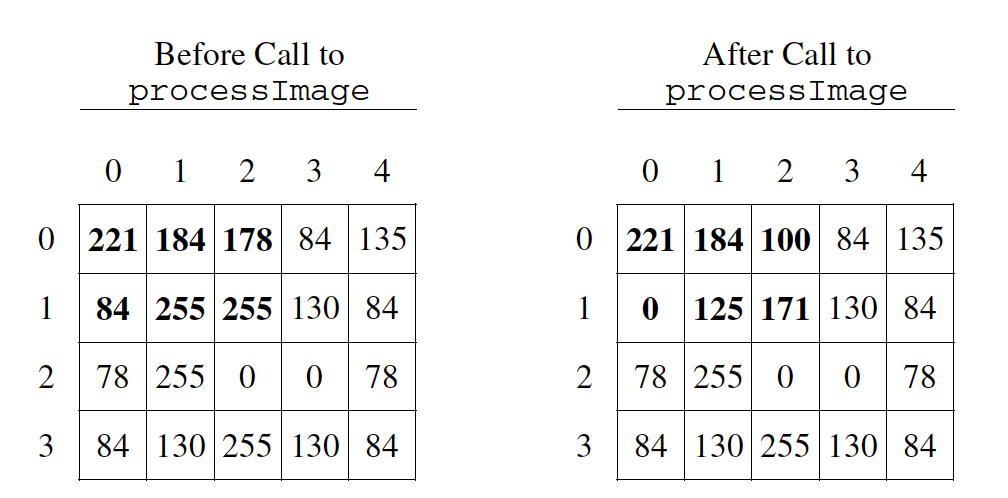# Free Response - Gray Image B¶

The following is part b of a free response question from 2012. It was question 4 on the exam. You can see all the free response questions from past exams at https://apstudents.collegeboard.org/courses/ap-computer-science-a/free-response-questions-by-year.

Question 4. A grayscale image is represented by a 2-dimensional rectangular array of pixels (picture elements). A pixel is an integer value that represents a shade of gray. In this question, pixel values can be in the range from 0 through 255, inclusive. A black pixel is represented by 0, and a white pixel is represented by 255. The declaration of the `GrayImage` class is shown below.

```public class GrayImage
{
public static final int BLACK = 0;
public static final int WHITE = 255;

/** The 2-dimensional representation of this image.
*  Guaranteed not to be null.
*  All values in the array are within the range
*  [BLACK, WHITE], inclusive.
*/
private int[][] pixelValues;

/** Processes this image in row-major order and
*  decreases the value of each pixel at position (row, col)
*  by the value of the pixel at position (row + 2, col + 2)
*  if it exists.
* Resulting values that would be less than BLACK are replaced
*   by BLACK.
* Pixels for which there is no pixel at
*   position (row + 2, col + 2) are unchanged.
*/
public void processImage()
{ /* to be implemented in part (b) */ }
}
```

Part b. Write the method `processImage` that modifies the image by changing the values in the instance variable `pixelValues` according to the following description. The pixels in the image are processed one at a time in row-major order. Row-major order processes the first row in the array from left to right and then processes the second row from left to right, continuing until all rows are processed from left to right. The first index of `pixelValues` represents the row number, and the second index represents the column number.

The pixel value at position (row, col) is decreased by the value at position (row + 2, col + 2) if such a position exists. If the result of the subtraction is less than the value `BLACK`, the pixel is assigned the value of `BLACK`. The values of the pixels for which there is no pixel at position (row + 2, col + 2) remain unchanged. You may assume that all the original values in the array are within the range [`BLACK`, `WHITE`], inclusive.

The following diagram shows the contents of the instance variable `pixelValues` before and after a call to `processImage`. The values shown in boldface represent the pixels that could be modified in a grayscale image with 4 rows and 5 columns.Figure 1: Example before and after a call to processImage

```public class GrayImage
{
public static final int BLACK = 0;
public static final int WHITE = 255;

/** The 2-dimensional representation of this image.
*  Guaranteed not to be null.
*  All values in the array are within the range
*  [BLACK, WHITE], inclusive.
*/
private int[][] pixelValues;

/** Processes this image in row-major order and
*  decreases the value of each pixel at position (row, col)
*  by the value of the pixel at position (row + 2, col + 2)
*  if it exists.
* Resulting values that would be less than BLACK are replaced
*   by BLACK.
* Pixels for which there is no pixel at
*   position (row + 2, col + 2) are unchanged.
*/
public void processImage()
{ /* to be implemented in part (b) */ }
}
```

## How to solve this problem¶

Once again, this problem starts with looping through the array of pixels, using a nested for loop for the 2D array. As we loop we will need to subtract pixel values from one another.

When comparing our pixel values to values deeper in the array, we need to be careful to correctly set the terminating conditions on the for loops. The code below prints out the pixel value at position (row, col) after it is decreased by the value at position (row + 2, col + 2), but what if (row+2,col+2) is beyond the array bounds? Can you fix the terminating conditions of the loops so that we do not go beyond the array bounds? You can step through the code using the Java Visualizer.

Try to write the code for the method `processImage`. When you are ready click “Run” to test your solution.

## Video - One way to code the solution¶

The following video is also on YouTube at https://youtu.be/8j34xQkjsJI. It walks through coding a solution.